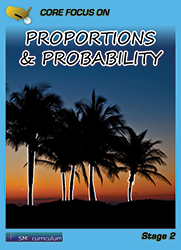#### Proportions & Probability

In this course, students analyze proportional relationships and use them to solve real-world and mathematical problems.  They use random sampling to draw inferences about a population.  And draw informal comparative inferences about two populations.  They investigate chance processes and develop, use, and evaluate probability models.

### Textbook & Stage I Series Pricing

1 FREE Teacher Edition(TE) and Teacher Resource Binder(TR) per 40 student texts ordered!